Next: Variational Principle Up: Identical Particles Previous: Helium Atom

# Orthohelium and Parahelium

Consider an excited state of the helium atom in which one electron is in the ground state, while the other is in a state characterized by the quantum numbers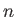,,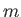, where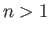. Making use of the Hamiltonian (9.52), and treating the final term as a perturbation, we can write the energy of this state as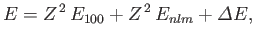(9.64)

where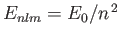is the energy of a hydrogen atom electron whose quantum numbers are,,,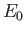the hydrogen ground-state energy, and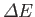the expectation value of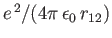. It follows from Equation (9.53) (with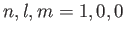and) that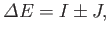(9.65)

where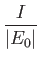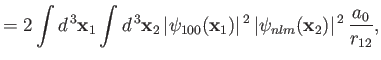(9.66)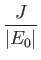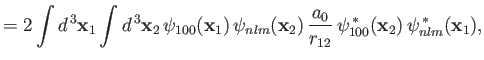(9.67)

and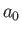is the Bohr radius. Here, the plus sign in Equation (9.66) corresponds to the spin-singlet state, whereas the minus sign corresponds to the spin-triplet state. The integral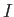--which is known as the direct integral--is obviously positive. The integral--which is known as the exchange integral--can be shown also to be positive. (See Exercises 13 and 14.) Hence, we conclude that, in excited states of helium, the spin-singlet state has a higher energy than the corresponding spin-triplet state. Incidentally, helium in the spin-singlet state is known as parahelium, whereas helium in the triplet state is called orthohelium. As we have seen, only parahelium is possible in the ground state,

The fact that parahelium energy levels lie slightly above corresponding orthohelium levels is interesting because our original Hamiltonian, (9.52), does not explicitly depend on spin. Nevertheless, there is a spin dependent effect--that is, a helium atom has a lower energy when its electrons possess parallel spins--as a consequence of Fermi-Dirac statistics. To be more exact, the energy is lower in the spin-triplet state because the corresponding spatial wavefunction is antisymmetric, causing the electrons to tend to avoid one another (thereby reducing their electrostatic repulsion).Next: Variational Principle Up: Identical Particles Previous: Helium Atom
Richard Fitzpatrick 2016-01-22